# How to Find the Earliest and Latest Date in Excel

We have a range of dates and we want to look up the earliest and the latest date based on certain criteria like the earliest date for a showing movie, we can use MIN and MAX functions with IF function or INDEX function together to find the matched date based on some criteria. Except using formula, we can also use PivotTable to find the min or max date.

See the table below. We have a list of entertainments in recent days. And we want to know the earliest date and latest date for certain entertainments.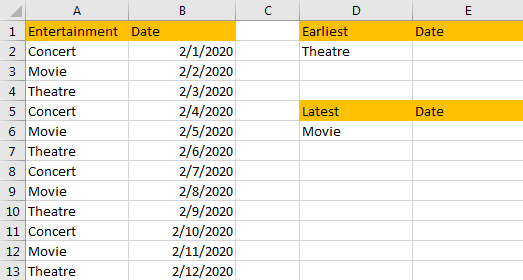## Find the Earliest Date Based on Criteria with Functions MIN & IF

Step 1: In E2 cell enter the formula =MIN(IF(\$A\$2:\$A\$13=D2,\$B\$2:\$B\$13)).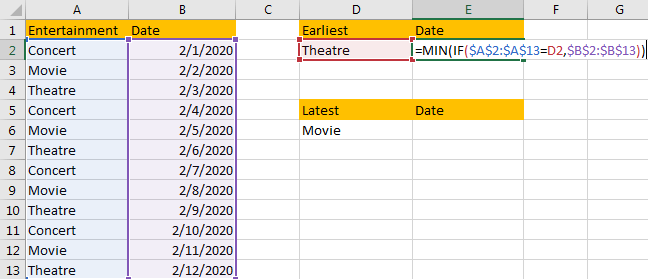Comment:

In above formula IF function returns the data satisfied the criteria \$A\$2:\$A\$13=D2, then MIN function returns the earliest date among the filtered dates.

Step 2: Press Ctrl+Shift+Enter to get the result. Verify that it returns a five digits number, so we need to convert it to date format.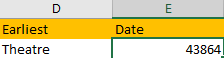Step 3: Click Home, in Number group, click General dropdown list and select Short Date.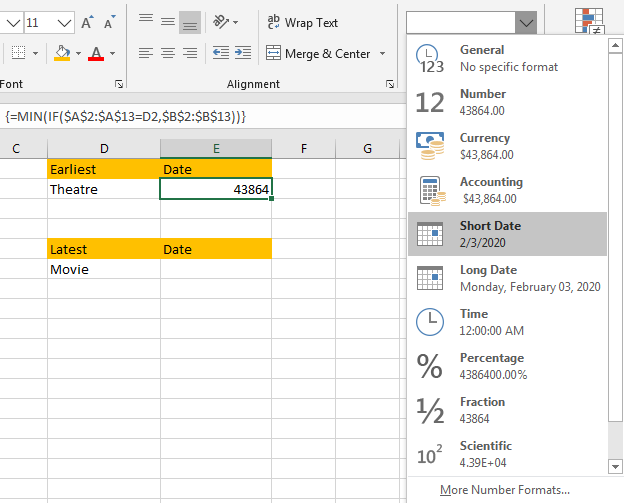Step 4: Verify that the five digits number is converted to date format properly.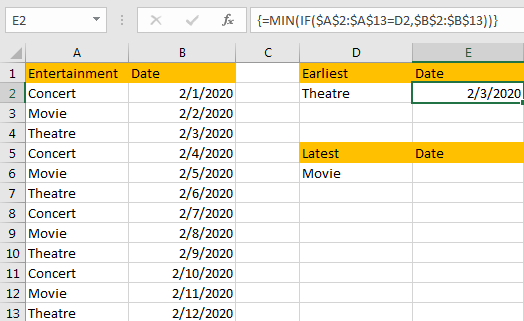## Find the Latest Date Based on Criteria with Functions MAX & IF

Step 1: In E6 cell enter the formula =MAX(IF(A2:A13=D6, B2:B13)).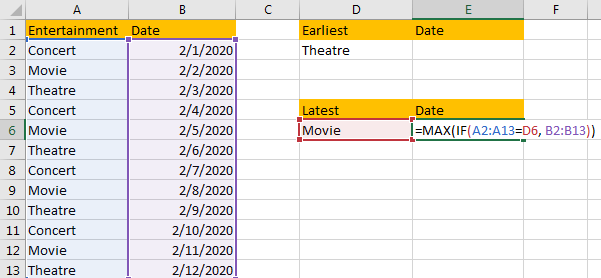Step 2: Press Ctrl+Shift+Enter to get the result. Verify that it returns a five digits number, so we need to convert it to date format.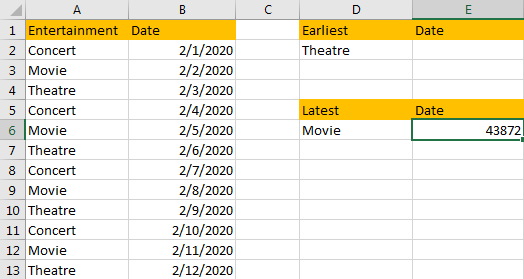Step 3: Click Home, in Number group, click General dropdown list and select Short Date. Verify that number is converted to date format properly.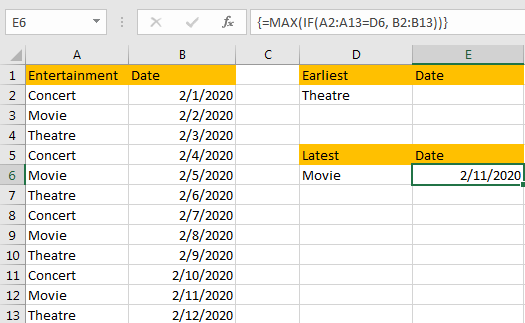There is another way to find the latest date by functions MIN and INDEX. See method below.

## Find the Latest Date Based on Criteria with Functions MIN & INDEX

Step 1: In E6 cell enter the formula =MAX(INDEX((D6=A2:A13)*B2:B13,)).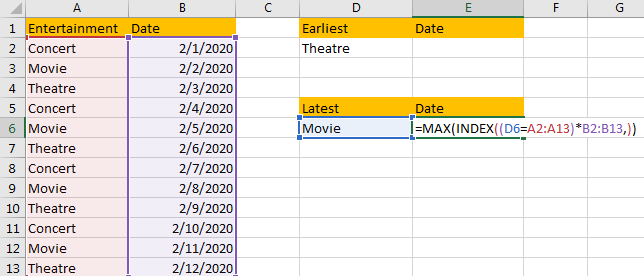Step 2: Press Ctrl+Shift+Enter to get the result. We get the same 5 digits number in above method step#2. The we can follow previous steps#3-#4 to convert it to date format.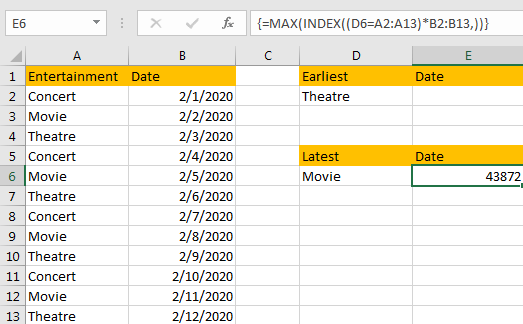### Related Functions

• Excel IF function
The Excel IF function perform a logical test to return one value if the condition is TRUE and return another value if the condition is FALSE. The IF function is a build-in function in Microsoft Excel and it is categorized as a Logical Function.The syntax of the IF function is as below:= IF (condition, [true_value], [false_value])….
• Excel INDEX function
The Excel INDEX function returns a value from a table based on the index (row number and column number)The INDEX function is a build-in function in Microsoft Excel and it is categorized as a Lookup and Reference Function.The syntax of the INDEX function is as below:= INDEX (array, row_num,[column_num])…
• Excel MAX function
The Excel MAX function returns the largest numeric value from the numbers that you provided. Or returns the largest value in the array.= MAX(num1,[num2,…numn])…
• Excel MIN function
The Excel MIN function returns the smallest numeric value from the numbers that you provided. Or returns the smallest value in the array.The MIN function is a build-in function in Microsoft Excel and it is categorized as a Statistical Function.The syntax of the MIN function is as below:= MIN(num1,[num2,…numn])….
Related Posts

Basic Array Formula With Examples

Are you searching for an article for getting the basic array formula with different examples for better understanding? Then congratulations because you have just landed on the right article. In this article, you would get to know the basic array ...

Calculate Average Of Last 5 Or N Values In Columns

Suppose you come across a task where you need to calculate the average of the last 2 or 3 numeric values, then what would you do? If you are new to Excel, then your first attempt might be doing this ...

Get Address of First Cell in Range

We will learn how to get address of first cell in range in this post. At Instance, we must determine the address of first cell in range to use as the data table's value. For the DATA table, we have ...

Extract Multiple Match Values into Separate Columns

If you have a few values/items in the excel sheet and you are thinking that with the aid of the “VlOOKUP” function you can look for a specific value, extract it and then put the matching item into the separate ...

How to Look Up the Lowest Value in A List by VLOOKUP/INDEX/MATCH Functions in Excel

VLOOKUP function is very useful in our daily work and we can use it to look up match value in a range, then get proper returned value (the returned value may be just adjacent to the match value). Sometimes we ...

How to Find the Smallest Value and Smallest Positive Value in Excel

Sometimes we want to find out the smallest positive value among a set of values. We should ignore the negative value and zero value when getting the smallest positive value. So we design different cases in this article to demonstrate ...

How to Calculate the Average Excluding the Smallest & Highest Numbers in Excel

Calculating the average for a batch of data is frequently used in our daily life. But for some cases like statistic the average score in a competition, or price analysis, we often calculate the average excluding the smallest and highest ...

How to Get the Maximum or Minimum Absolute Value in Excel

It is easy to find the maximum or minimum value in a batch of data in excel, but if this batch of numbers contains both positive and negative numbers, the maximum or minimum absolute value cannot be found out by ...

How to Find Max And Min Value with Single or Multiple Criteria in Excel

This post will guide you how to find the maximum value in a range of cells based on single or multiple criteria in Excel. How do I calculate the Minimum value based on criteria with MIN Function in Excel. Find ...

How to Check If value Is between Two Numbers in Excel

This post will guide you how to check if a cell value is between two numbers in Excel. How do I build an IF statement that test if a cell value is between two given values in formula in Excel. ...

Sidebar